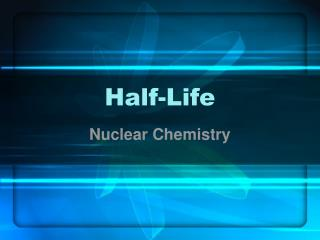DownloadDownload PresentationHalf-Life

# Half-Life

Télécharger la présentation## Half-Life

- - - - - - - - - - - - - - - - - - - - - - - - - - - E N D - - - - - - - - - - - - - - - - - - - - - - - - - - -
##### Presentation Transcript

1. Half-Life Nuclear Chemistry

2. What is Half-Life? • The time it takes for half of a given amount of a radioactive isotope to undergo decay.

3. Note: The time for the 1st half life is the same as the time for the 2nd half life ……. Same isotopes 1st Half-Life = decay time to divide in half 1/2 2nd Half-Life = decay time to divide in half 1/4

4. QUESTION? Does decay slow down, speed up, or remain constant after each half-life? Half-life equal in time 1st Half-Life 2nd Half-Life Decay slows down If you have to eat ½ in the same amount of time – you have to eat slower.

5. What is Half–Life Used For ? • Tells the age of a fossil • Carbon-14 Dating How many years ago did the volcano erupt? 6.25 % of Carbon-14

6. FACT:Carbon – 14 has a half-life of 5730 years 1 1/2 .5 = 50% 2 ½ x ½ = 1/4 .25 = 25% 3 1/4 x ½ = 1/8 .125 = 12.5% 4 4 1/8 x 1/2 = 1/16 . 0625 = 6.25% . 0625 = 6.25% 4 x 5730 = 22,920 years

7. A rock with 25% of potassium-40 was analyzed. How long ago was the rock formed? 1.25 billion years How many half-life does it take to get 25% of potassium-40? Potassium – 40 half-life? 1 1/2 .5 = 50% 2 2 ½ x ½ = 1/4 .25 = 25% 2 x 1.25 billion years – 2.5 billions years

8. Ash from a fire pit was found to have 12.5 % as much carbon – 14 as would be found in ash today. How long ago was the ash formed? How many half-life does it take to get 12.5% of Carbon-14? 5730 years Carbon-14 half-life? 1 1/2 .5 = 50% 2 ½ x ½ = 1/4 .25 = 25% 3 1/4 x ½ = 1/8 3 3 x 5730 years = 17, 190 years

9. The half-life of radon-22 is 3.8 days. How much of a 100 g sample is left after 15.2 days? 100g 1 3.8 ½ of 100 = 50 g ½ of 50 or ¼ of 100 = 25g 2 7.6 ½ of 25 or 1/8 of 100 = 12.5g 11.4 3 ½ of 12.5 or 1/16 or 100 = 6.25g 4 15,.2 Notice: Total number of days/ half-life days= number of half-lives

10. Carbon-14 has a half-life of 5,730 years. If a sample contains 70 mg originally, how much is left after 17,190 years? 70g 1 ½ of 70 = 35 mg 5,730 2 ½ of 35 or ¼ of 70 = 17.5 mg 11,460 ½ of 17.5 or 1/8 of 70 = 8.75 mg 3 17,190 Mathematical Formula: Total number of days/ half-life days= number of half-lives 17190/5730 = 3 1/8 of original 1/8 x 70 = 8.75 mg